Chapter 6. Electronic Structure and Periodic Properties of Elements

# 6.3 Development of Quantum Theory

### Learning Objectives

By the end of this section, you will be able to:

• Extend the concept of wave–particle duality that was observed in electromagnetic radiation to matter as well
• Understand the general idea of the quantum mechanical description of electrons in an atom, and that it uses the notion of three-dimensional wave functions, or orbitals, that define the distribution of probability to find an electron in a particular part of space
• List and describe traits of the four quantum numbers that form the basis for completely specifying the state of an electron in an atom

Bohr’s model explained the experimental data for the hydrogen atom and was widely accepted, but it also raised many questions. Why did electrons orbit at only fixed distances defined by a single quantum number n = 1, 2, 3, and so on, but never in between? Why did the model work so well describing hydrogen and one-electron ions, but could not correctly predict the emission spectrum for helium or any larger atoms? To answer these questions, scientists needed to completely revise the way they thought about matter.

# Behavior in the Microscopic World

We know how matter behaves in the macroscopic world—objects that are large enough to be seen by the naked eye follow the rules of classical physics. A billiard ball moving on a table will behave like a particle: It will continue in a straight line unless it collides with another ball or the table cushion, or is acted on by some other force (such as friction). The ball has a well-defined position and velocity (or a well-defined momentum, p = mv, defined by mass m and velocity v) at any given moment. In other words, the ball is moving in a classical trajectory. This is the typical behavior of a classical object.

When waves interact with each other, they show interference patterns that are not displayed by macroscopic particles such as the billiard ball. For example, interacting waves on the surface of water can produce interference patters similar to those shown on Figure 1. This is a case of wave behavior on the macroscopic scale, and it is clear that particles and waves are very different phenomena in the macroscopic realm.Figure 1. An interference pattern on the water surface is formed by interacting waves. The waves are caused by reflection of water from the rocks. (credit: modification of work by Sukanto Debnath)

As technological improvements allowed scientists to probe the microscopic world in greater detail, it became increasingly clear by the 1920s that very small pieces of matter follow a different set of rules from those we observe for large objects. The unquestionable separation of waves and particles was no longer the case for the microscopic world.

One of the first people to pay attention to the special behavior of the microscopic world was Louis de Broglie. He asked the question: If electromagnetic radiation can have particle-like character, can electrons and other submicroscopic particles exhibit wavelike character? In his 1925 doctoral dissertation, de Broglie extended the wave–particle duality of light that Einstein used to resolve the photoelectric-effect paradox to material particles. He predicted that a particle with mass m and velocity v (that is, with linear momentum p) should also exhibit the behavior of a wave with a wavelength value λ, given by this expression in which h is the familiar Planck’s constant:$\lambda = \frac{h}{mv} = \frac{h}{p}$

This is called the de Broglie wavelength. Unlike the other values of λ discussed in this chapter, the de Broglie wavelength is a characteristic of particles and other bodies, not electromagnetic radiation (note that this equation involves velocity [v, m/s], not frequency [ν, Hz]. Although these two symbols are identical, they mean very different things). Where Bohr had postulated the electron as being a particle orbiting the nucleus in quantized orbits, de Broglie argued that Bohr’s assumption of quantization can be explained if the electron is considered not as a particle, but rather as a circular standing wave such that only an integer number of wavelengths could fit exactly within the orbit (Figure 2).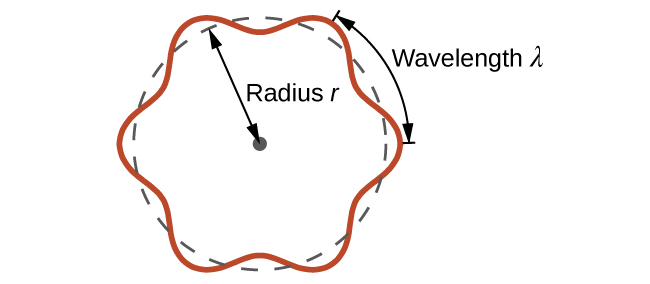Figure 2. If an electron is viewed as a wave circling around the nucleus, an integer number of wavelengths must fit into the orbit for this standing wave behavior to be possible.

For a circular orbit of radius r, the circumference is 2πr, and so de Broglie’s condition is:$2\pi r = n\lambda , n = 1, 2, 3, \cdots$

Since the de Broglie expression relates the wavelength to the momentum and, hence, velocity, this implies:$2 \pi r = n\lambda = \frac{nh}{p} = \frac{nh}{mv} = \frac{nhr}{mvr} = \frac{nhr}{L}$

This expression can be rearranged to give Bohr’s formula for the quantization of the angular momentum:$L = \frac{nh}{2\pi} = n{\hbar}$

Classical angular momentum L for a circular motion is equal to the product of the radius of the circle and the momentum of the moving particle p.$L = rp = rmv \;\text{(for a circular motion)}$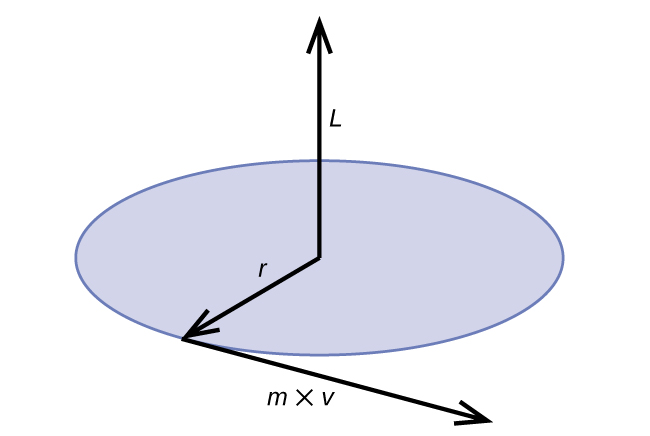Figure 3. The diagram shows angular momentum for a circular motion.

Shortly after de Broglie proposed the wave nature of matter, two scientists at Bell Laboratories, C. J. Davisson and L. H. Germer, demonstrated experimentally that electrons can exhibit wavelike behavior by showing an interference pattern for electrons travelling through a regular atomic pattern in a crystal. The regularly spaced atomic layers served as slits, as used in other interference experiments. Since the spacing between the layers serving as slits needs to be similar in size to the wavelength of the tested wave for an interference pattern to form, Davisson and Germer used a crystalline nickel target for their “slits,” since the spacing of the atoms within the lattice was approximately the same as the de Broglie wavelengths of the electrons that they used. Figure 4 shows an interference pattern. It is strikingly similar to the interference patterns for light shown in Figure 5 in Chapter 6.1 Electromagnetic Energy. The wave–particle duality of matter can be seen in Figure 4 by observing what happens if electron collisions are recorded over a long period of time. Initially, when only a few electrons have been recorded, they show clear particle-like behavior, having arrived in small localized packets that appear to be random. As more and more electrons arrived and were recorded, a clear interference pattern that is the hallmark of wavelike behavior emerged. Thus, it appears that while electrons are small localized particles, their motion does not follow the equations of motion implied by classical mechanics, but instead it is governed by some type of a wave equation that governs a probability distribution even for a single electron’s motion. Thus the wave–particle duality first observed with photons is actually a fundamental behavior intrinsic to all quantum particles.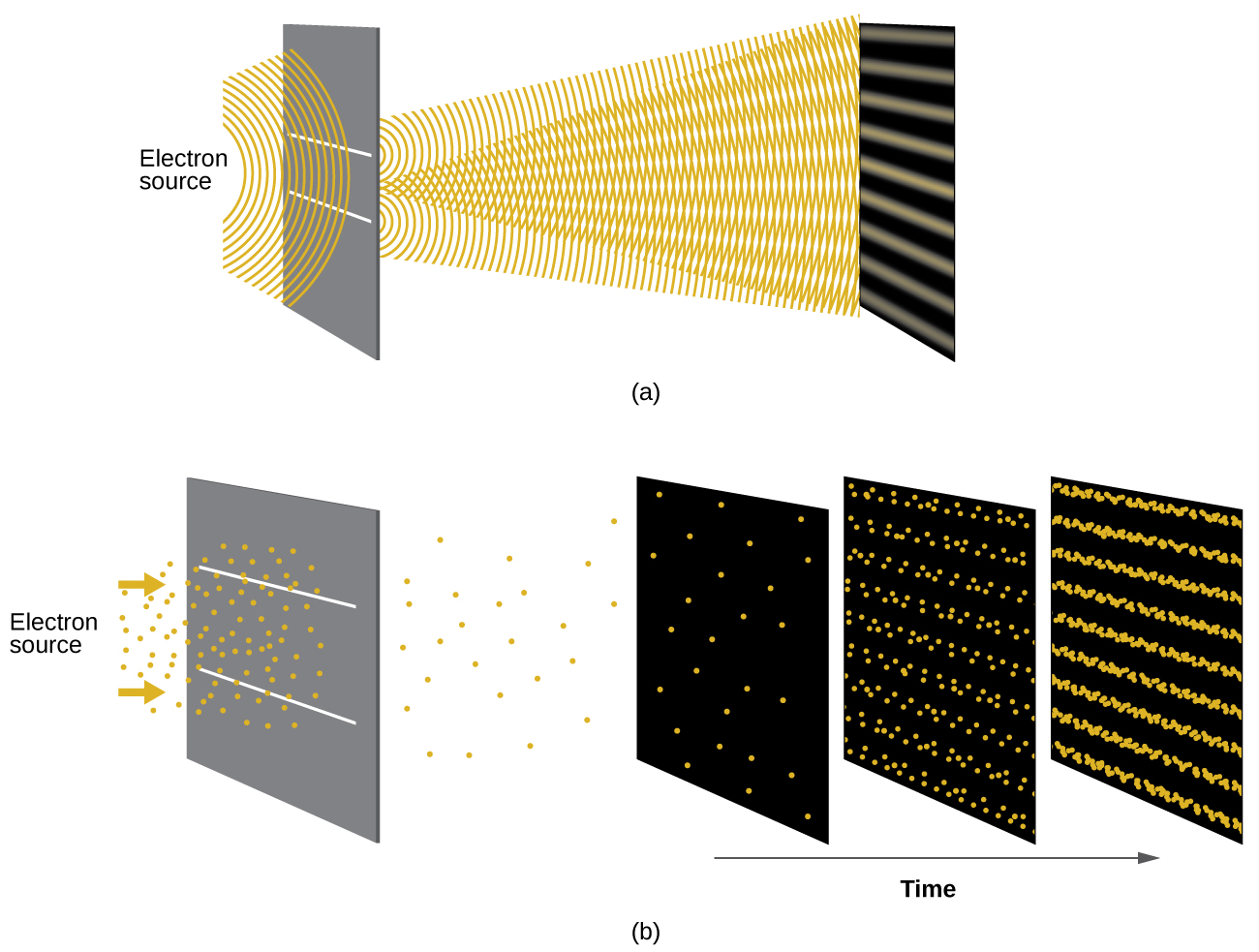Figure 4. (a) The interference pattern for electrons passing through very closely spaced slits demonstrates that quantum particles such as electrons can exhibit wavelike behavior. (b) The experimental results illustrated here demonstrate the wave–particle duality in electrons. The electrons pass through very closely spaced slits, forming an interference pattern, with increasing numbers of electrons being recorded from the left image to the right. With only a few electrons recorded, it is clear that the electrons arrive as individual localized “particles,” but in a seemingly random pattern. As more electrons arrive, a wavelike interference pattern begins to emerge. Note that the probability of the final electron location is still governed by the wave-type distribution, even for a single electron, but it can be observed more easily if many electron collisions have been recorded.View the Dr. Quantum – Double Slit Experiment cartoon for an easy-to-understand description of wave–particle duality and the associated experiments.

### Example 1

Calculating the Wavelength of a Particle
If an electron travels at a velocity of 1.000 × 107 m s–1 and has a mass of 9.109 × 10–28 g, what is its wavelength?

Solution
We can use de Broglie’s equation to solve this problem, but we first must do a unit conversion of Planck’s constant. You learned earlier that 1 J = 1 kg m2/s2. Thus, we can write h = 6.626 × 10–34 J s as 6.626 × 10–34 kg m2/s.$\lambda = \frac{h}{mv}$$\begin{array}{l} = \frac{6.626 \times 10^{-34} \;\text{kg m}^2 \text{/s}}{(9.190 \times 10^{-31} \;\text{kg})(1.000 \times 10^7 \;\text{m/s})} \\[1em] = 7.274 \times 10^{-11} \;\text{m} \end{array}$

This is a small value, but it is significantly larger than the size of an electron in the classical (particle) view. This size is the same order of magnitude as the size of an atom. This means that electron wavelike behavior is going to be noticeable in an atom.

Check Your Learning
Calculate the wavelength of a softball with a mass of 100 g traveling at a velocity of 35 m s–1, assuming that it can be modeled as a single particle.

### Answer:

1.9 × 10–34 m.

We never think of a thrown softball having a wavelength, since this wavelength is so small it is impossible for our senses or any known instrument to detect (strictly speaking, the wavelength of a real baseball would correspond to the wavelengths of its constituent atoms and molecules, which, while much larger than this value, would still be microscopically tiny). The de Broglie wavelength is only appreciable for matter that has a very small mass and/or a very high velocity.

Werner Heisenberg considered the limits of how accurately we can measure properties of an electron or other microscopic particles. He determined that there is a fundamental limit to how accurately one can measure both a particle’s position and its momentum simultaneously. The more accurately we measure the momentum of a particle, the less accurately we can determine its position at that time, and vice versa. This is summed up in what we now call the Heisenberg uncertainty principle: It is fundamentally impossible to determine simultaneously and exactly both the momentum and the position of a particle. For a particle of mass m moving with velocity vx in the x direction (or equivalently with momentum px), the product of the uncertainty in the position, Δx, and the uncertainty in the momentum, Δpx , must be greater than or equal to$\frac{\hbar}{2}$ (recall that$\hbar = \frac{h}{2 \pi}$, the value of Planck’s constant divided by 2π).$\Delta x \times \Delta p_x = (\Delta x)(m \Delta v) \ge \frac{\hbar}{2}$

This equation allows us to calculate the limit to how precisely we can know both the simultaneous position of an object and its momentum. For example, if we improve our measurement of an electron’s position so that the uncertainty in the position (Δx) has a value of, say, 1 pm (10–12 m, about 1% of the diameter of a hydrogen atom), then our determination of its momentum must have an uncertainty with a value of at least$[\Delta p = m\Delta v = \frac{h}{(2 \Delta x )}] = \frac{(1.055 \times 10^{-34} \;\text{kg m}^2/\text{s})}{(2 \times 1 \times 10^{-12} \;\text{m})} = 5 \times 10^{-23} \;\text{kg m/s}$

The value of ħ is not large, so the uncertainty in the position or momentum of a macroscopic object like a baseball is too insignificant to observe. However, the mass of a microscopic object such as an electron is small enough that the uncertainty can be large and significant.

It should be noted that Heisenberg’s uncertainty principle is not just limited to uncertainties in position and momentum, but it also links other dynamical variables. For example, when an atom absorbs a photon and makes a transition from one energy state to another, the uncertainty in the energy and the uncertainty in the time required for the transition are similarly related, as ΔE Δt$\Delta E \; \Delta t \ge \frac{\hbar}{2}$. As will be discussed later, even the vector components of angular momentum cannot all be specified exactly simultaneously.

Heisenberg’s principle imposes ultimate limits on what is knowable in science. The uncertainty principle can be shown to be a consequence of wave–particle duality, which lies at the heart of what distinguishes modern quantum theory from classical mechanics. Recall that the equations of motion obtained from classical mechanics are trajectories where, at any given instant in time, both the position and the momentum of a particle can be determined exactly. Heisenberg’s uncertainty principle implies that such a view is untenable in the microscopic domain and that there are fundamental limitations governing the motion of quantum particles. This does not mean that microscopic particles do not move in trajectories, it is just that measurements of trajectories are limited in their precision. In the realm of quantum mechanics, measurements introduce changes into the system that is being observed.Read this article that describes a recent macroscopic demonstration of the uncertainty principle applied to microscopic objects.

# The Quantum–Mechanical Model of an Atom

Shortly after de Broglie published his ideas that the electron in a hydrogen atom could be better thought of as being a circular standing wave instead of a particle moving in quantized circular orbits, as Bohr had argued, Erwin Schrödinger extended de Broglie’s work by incorporating the de Broglie relation into a wave equation, deriving what is today known as the Schrödinger equation. When Schrödinger applied his equation to hydrogen-like atoms, he was able to reproduce Bohr’s expression for the energy and, thus, the Rydberg formula governing hydrogen spectra, and he did so without having to invoke Bohr’s assumptions of stationary states and quantized orbits, angular momenta, and energies; quantization in Schrödinger’s theory was a natural consequence of the underlying mathematics of the wave equation. Like de Broglie, Schrödinger initially viewed the electron in hydrogen as being a physical wave instead of a particle, but where de Broglie thought of the electron in terms of circular stationary waves, Schrödinger properly thought in terms of three-dimensional stationary waves, or wavefunctions, represented by the Greek letter psi, ψ. A few years later, Max Born proposed an interpretation of the wavefunction ψ that is still accepted today: Electrons are still particles, and so the waves represented by ψ are not physical waves but, instead, are complex probability amplitudes. The square of the magnitude of a wavefunction$|\psi|^2$ describes the probability of the quantum particle being present near a certain location in space. This means that wavefunctions can be used to determine the distribution of the electron’s density with respect to the nucleus in an atom. In the most general form, the Schrödinger equation can be written as:$\hat{\mathcal{H}} \psi = E \psi$$\hat{\mathcal{H}}$ is the Hamiltonian operator, a set of mathematical operations representing the total energy of the quantum particle (such as an electron in an atom), ψ is the wavefunction of this particle that can be used to find the special distribution of the probability of finding the particle, and$E$ is the actual value of the total energy of the particle.

Schrödinger’s work, as well as that of Heisenberg and many other scientists following in their footsteps, is generally referred to as quantum mechanics.You may also have heard of Schrödinger because of his famous thought experiment. This story explains the concepts of superposition and entanglement as related to a cat in a box with poison.

# Understanding Quantum Theory of Electrons in Atoms

The goal of this section is to understand the electron orbitals (location of electrons in atoms), their different energies, and other properties. The use of quantum theory provides the best understanding to these topics. This knowledge is a precursor to chemical bonding.

As was described previously, electrons in atoms can exist only on discrete energy levels but not between them. It is said that the energy of an electron in an atom is quantized, that is, it can be equal only to certain specific values and can jump from one energy level to another but not transition smoothly or stay between these levels.

The energy levels are labeled with an n value, where n = 1, 2, 3, …. Generally speaking, the energy of an electron in an atom is greater for greater values of n. This number, n, is referred to as the principle quantum number. The principle quantum number defines the location of the energy level. It is essentially the same concept as the n in the Bohr atom description. Another name for the principal quantum number is the shell number. The shells of an atom can be thought of concentric circles radiating out from the nucleus. The electrons that belong to a specific shell are most likely to be found within the corresponding circular area. The further we proceed from the nucleus, the higher the shell number, and so the higher the energy level (Figure 5). The positively charged protons in the nucleus stabilize the electronic orbitals by electrostatic attraction between the positive charges of the protons and the negative charges of the electrons. So the further away the electron is from the nucleus, the greater the energy it has.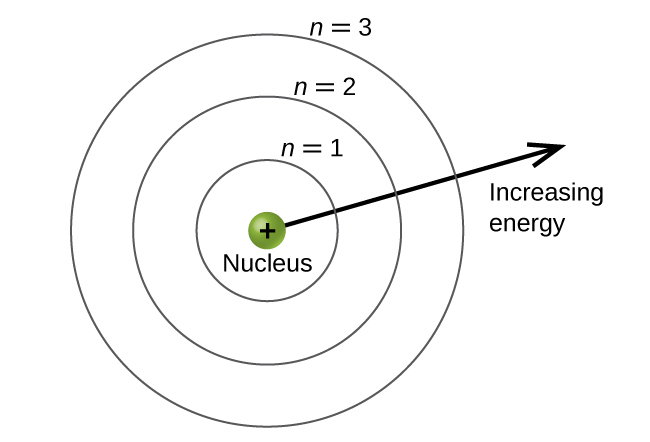Figure 5. Different shells are numbered by principle quantum numbers.

This quantum mechanical model for where electrons reside in an atom can be used to look at electronic transitions, the events when an electron moves from one energy level to another. If the transition is to a higher energy level, energy is absorbed, and the energy change has a positive value. To obtain the amount of energy necessary for the transition to a higher energy level, a photon is absorbed by the atom. A transition to a lower energy level involves a release of energy, and the energy change is negative. This process is accompanied by emission of a photon by the atom. The following equation summarizes these relationships and is based on the hydrogen atom:$\Delta E = E_{\text{final}} - E_{\text{initial}} \\ [1em] = -2.18 \times 10^{-18} (\frac{1}{n^2_\text{f}} - \frac{1}{n^2_\text{i}}) \;\text{J}$

The values nf and ni are the final and initial energy states of the electron. Example 2 in Chapter 6.2 The Bohr Model in the previous section of the chapter demonstrates calculations of such energy changes.

The principal quantum number is one of three quantum numbers used to characterize an orbital. An atomic orbital, which is distinct from an orbit, is a general region in an atom within which an electron is most probable to reside. The quantum mechanical model specifies the probability of finding an electron in the three-dimensional space around the nucleus and is based on solutions of the Schrödinger equation. In addition, the principle quantum number defines the energy of an electron in a hydrogen or hydrogen-like atom or an ion (an atom or an ion with only one electron) and the general region in which discrete energy levels of electrons in a multi-electron atoms and ions are located.

Another quantum number is l, the angular momentum quantum number. It is an integer that defines the shape of the orbital, and takes on the values, l = 0, 1, 2, …, n – 1. This means that an orbital with n = 1 can have only one value of l, l = 0, whereas n = 2 permits l = 0 and l = 1, and so on. The principal quantum number defines the general size and energy of the orbital. The l value specifies the shape of the orbital. Orbitals with the same value of l form a subshell. In addition, the greater the angular momentum quantum number, the greater is the angular momentum of an electron at this orbital.

Orbitals with l = 0 are called s orbitals (or the s subshells). The value l = 1 corresponds to the p orbitals. For a given n, p orbitals constitute a p subshell (e.g., 3p if n = 3). The orbitals with l = 2 are called the d orbitals, followed by the f-, g-, and h-orbitals for l = 3, 4, 5, and there are higher values we will not consider.

There are certain distances from the nucleus at which the probability density of finding an electron located at a particular orbital is zero. In other words, the value of the wavefunction ψ is zero at this distance for this orbital. Such a value of radius r is called a radial node. The number of radial nodes in an orbital is nl – 1.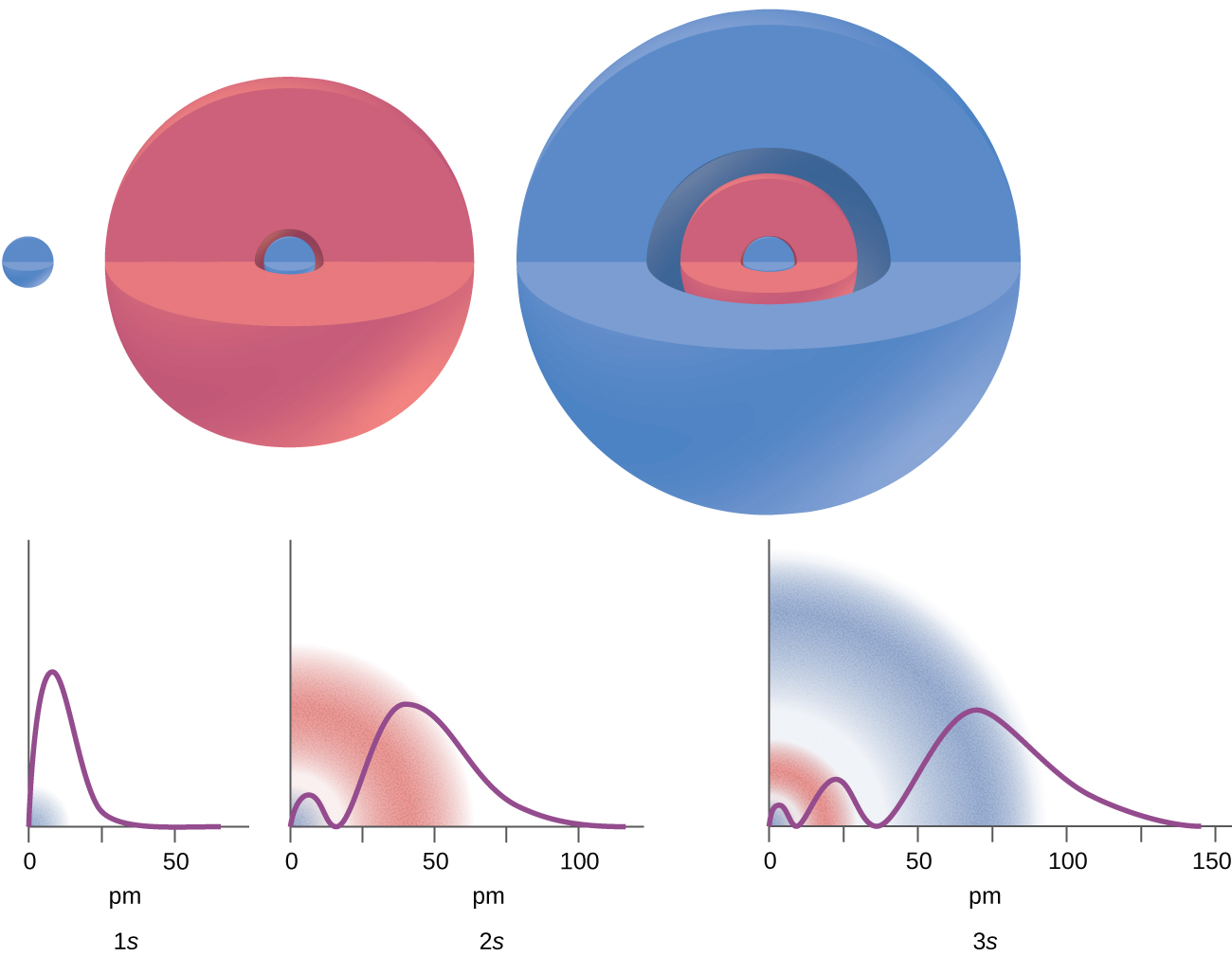Figure 6. The graphs show the probability (y axis) of finding an electron for the 1s, 2s, 3s orbitals as a function of distance from the nucleus.

Consider the examples in Figure 6. The orbitals depicted are of the s type, thus l = 0 for all of them. It can be seen from the graphs of the probability densities that there are 1 – 0 – 1 = 0 places where the density is zero (nodes) for 1s (n = 1), 2 – 0 – 1 = 1 node for 2s, and 3 – 0 – 1 = 2 nodes for the 3s orbitals.

The s subshell electron density distribution is spherical and the p subshell has a dumbbell shape. The d and f orbitals are more complex. These shapes represent the three-dimensional regions within which the electron is likely to be found.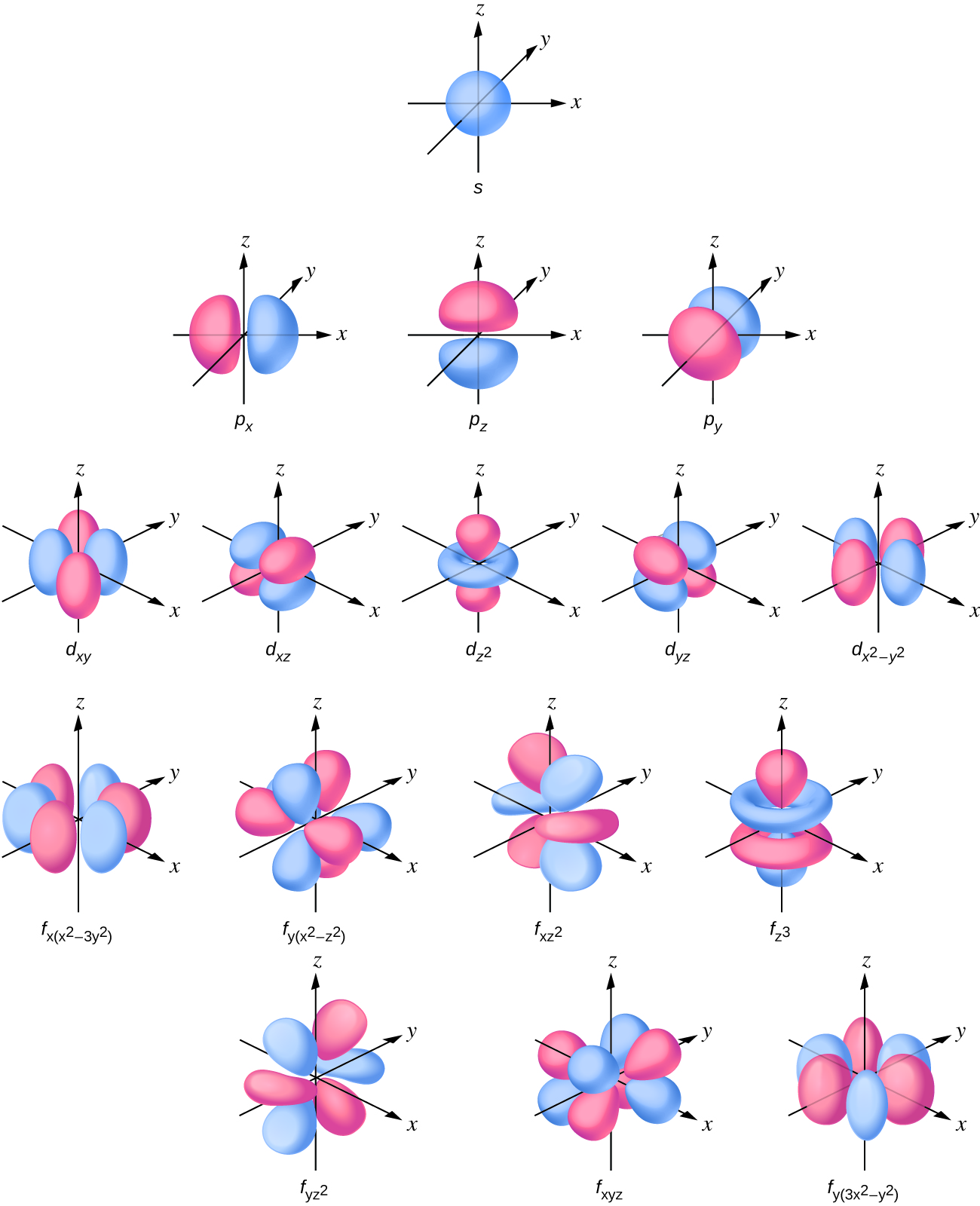Figure 7. Shapes of s, p, d, and f orbitals.

If an electron has an angular momentum (l ≠ 0), then this vector can point in different directions. In addition, the z component of the angular momentum can have more than one value. This means that if a magnetic field is applied in the z direction, orbitals with different values of the z component of the angular momentum will have different energies resulting from interacting with the field. The magnetic quantum number, called ml, specifies the z component of the angular momentum for a particular orbital. For example, for an s orbital, l = 0, and the only value of ml is zero. For p orbitals, l = 1, and ml can be equal to –1, 0, or +1. Generally speaking, ml can be equal to –l, –(l – 1), …, –1, 0, +1, …, (l – 1), l. The total number of possible orbitals with the same value of l (a subshell) is 2l + 1. Thus, there is one s-orbital for ml = 0, there are three p-orbitals for ml = 1, five d-orbitals for ml = 2, seven f-orbitals for ml = 3, and so forth. The principle quantum number defines the general value of the electronic energy. The angular momentum quantum number determines the shape of the orbital. And the magnetic quantum number specifies orientation of the orbital in space, as can be seen in Figure 7.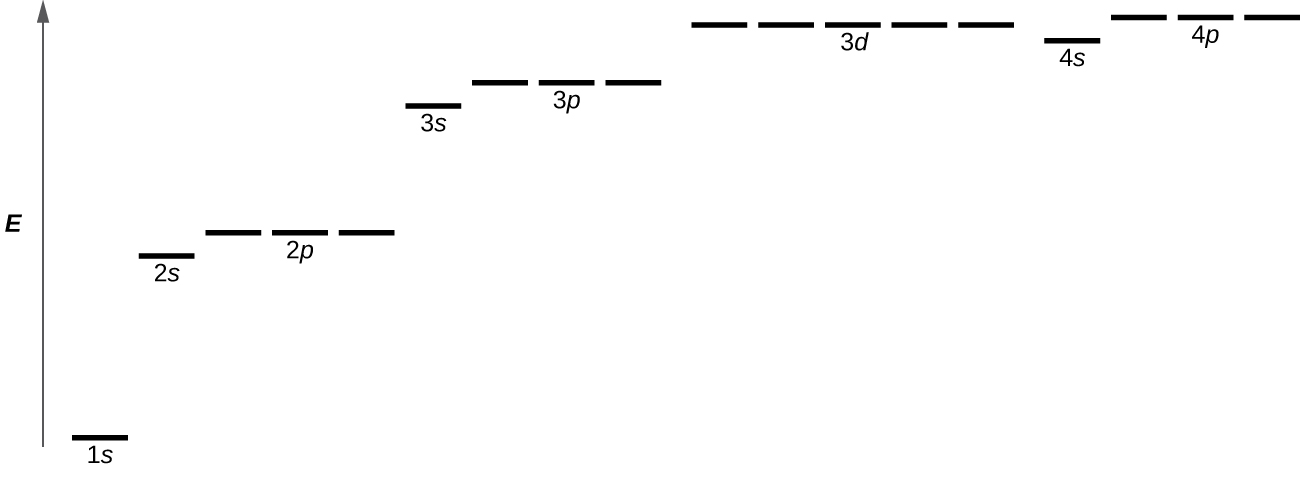Figure 8. The chart shows the energies of electron orbitals in a multi-electron atom.

Figure 8 illustrates the energy levels for various orbitals. The number before the orbital name (such as 2s, 3p, and so forth) stands for the principle quantum number, n. The letter in the orbital name defines the subshell with a specific angular momentum quantum number l = 0 for s orbitals, 1 for p orbitals, 2 for d orbitals. Finally, there are more than one possible orbitals for l ≥ 1, each corresponding to a specific value of ml. In the case of a hydrogen atom or a one-electron ion (such as He+, Li2+, and so on), energies of all the orbitals with the same n are the same. This is called a degeneracy, and the energy levels for the same principle quantum number, n, are called degenerate energy levels. However, in atoms with more than one electron, this degeneracy is eliminated by the electron–electron interactions, and orbitals that belong to different subshells have different energies, as shown on Figure 8. Orbitals within the same subshell (for example ns, np, nd, nf, such as 2p, 3s) are still degenerate and have the same energy.

While the three quantum numbers discussed in the previous paragraphs work well for describing electron orbitals, some experiments showed that they were not sufficient to explain all observed results. It was demonstrated in the 1920s that when hydrogen-line spectra are examined at extremely high resolution, some lines are actually not single peaks but, rather, pairs of closely spaced lines. This is the so-called fine structure of the spectrum, and it implies that there are additional small differences in energies of electrons even when they are located in the same orbital. These observations led Samuel Goudsmit and George Uhlenbeck to propose that electrons have a fourth quantum number. They called this the spin quantum number, or ms.

The other three quantum numbers, n, l, and ml, are properties of specific atomic orbitals that also define in what part of the space an electron is most likely to be located. Orbitals are a result of solving the Schrödinger equation for electrons in atoms. The electron spin is a different kind of property. It is a completely quantum phenomenon with no analogues in the classical realm. In addition, it cannot be derived from solving the Schrödinger equation and is not related to the normal spatial coordinates (such as the Cartesian x, y, and z). Electron spin describes an intrinsic electron “rotation” or “spinning.” Each electron acts as a tiny magnet or a tiny rotating object with an angular momentum, even though this rotation cannot be observed in terms of the spatial coordinates.

The magnitude of the overall electron spin can only have one value, and an electron can only “spin” in one of two quantized states. One is termed the α state, with the z component of the spin being in the positive direction of the z axis. This corresponds to the spin quantum number$m_s = \frac{1}{2}$. The other is called the β state, with the z component of the spin being negative and$m_s = -\frac{1}{2}$. Any electron, regardless of the atomic orbital it is located in, can only have one of those two values of the spin quantum number. The energies of electrons having$m_s = - \frac{1}{2}$ and$m_s = \frac{1}{2}$ are different if an external magnetic field is applied.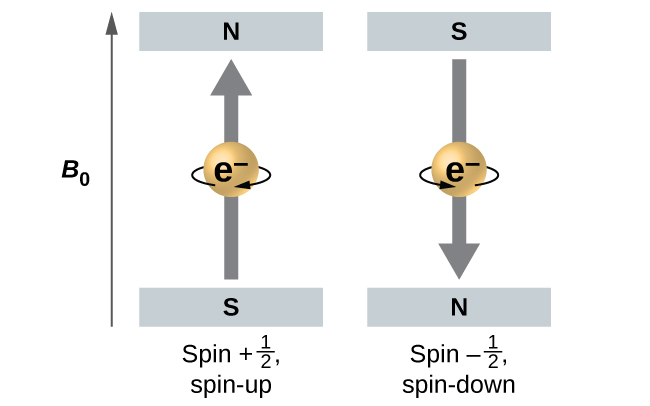Figure 9. Electrons with spin values ±1/2 in an external magnetic field.

Figure 9 illustrates this phenomenon. An electron acts like a tiny magnet. Its moment is directed up (in the positive direction of the z axis) for the$\frac{1}{2}$ spin quantum number and down (in the negative z direction) for the spin quantum number of$- \frac{1}{2}$. A magnet has a lower energy if its magnetic moment is aligned with the external magnetic field (the left electron on Figure 9) and a higher energy for the magnetic moment being opposite to the applied field. This is why an electron with$m_s = \frac{1}{2}$ has a slightly lower energy in an external field in the positive z direction, and an electron with$m_s = -\frac{1}{2}$ has a slightly higher energy in the same field. This is true even for an electron occupying the same orbital in an atom. A spectral line corresponding to a transition for electrons from the same orbital but with different spin quantum numbers has two possible values of energy; thus, the line in the spectrum will show a fine structure splitting.

# The Pauli Exclusion Principle

An electron in an atom is completely described by four quantum numbers: n, l, ml, and ms. The first three quantum numbers define the orbital and the fourth quantum number describes the intrinsic electron property called spin. An Austrian physicist Wolfgang Pauli formulated a general principle that gives the last piece of information that we need to understand the general behavior of electrons in atoms. The Pauli exclusion principle can be formulated as follows: No two electrons in the same atom can have exactly the same set of all the four quantum numbers. What this means is that electrons can share the same orbital (the same set of the quantum numbers n, l, and ml), but only if their spin quantum numbers ms have different values. Since the spin quantum number can only have two values ($\pm \frac{1}{2}$), no more than two electrons can occupy the same orbital (and if two electrons are located in the same orbital, they must have opposite spins). Therefore, any atomic orbital can be populated by only zero, one, or two electrons.

The properties and meaning of the quantum numbers of electrons in atoms are briefly summarized in Table 1.

Name Symbol Allowed values Physical meaning
principle quantum number n 1, 2, 3, 4, …. shell, the general region for the value of energy for an electron on the orbital
angular momentum or azimuthal quantum number l 0 ≤ ln – 1 subshell, the shape of the orbital
magnetic quantum number ml lmll orientation of the orbital
spin quantum number ms 12,−1212,−12 direction of the intrinsic quantum “spinning” of the electron
Table 1. Quantum Numbers, Their Properties, and Significance

### Example 2

Working with Shells and Subshells
Indicate the number of subshells, the number of orbitals in each subshell, and the values of l and ml for the orbitals in the n = 4 shell of an atom.

Solution
For n = 4, l can have values of 0, 1, 2, and 3. Thus, s, p, d, and f subshells are found in the n = 4 shell of an atom. For l = 0 (the s subshell), ml can only be 0. Thus, there is only one 4s orbital. For l = 1 (p-type orbitals), m can have values of –1, 0, +1, so we find three 4p orbitals. For l = 2 (d-type orbitals), ml can have values of –2, –1, 0, +1, +2, so we have five 4d orbitals. When l = 3 (f-type orbitals), ml can have values of –3, –2, –1, 0, +1, +2, +3, and we can have seven 4f orbitals. Thus, we find a total of 16 orbitals in the n = 4 shell of an atom.

Check Your Learning
Identify the subshell in which electrons with the following quantum numbers are found: (a) n = 3, l = 1; (b) n = 5, l = 3; (c) n = 2, l = 0.

### Answer:

(a) 3p (b) 5f (c) 2s

### Example 3

Maximum Number of Electrons
Calculate the maximum number of electrons that can occupy a shell with (a) n = 2, (b) n = 5, and (c) n as a variable. Note you are only looking at the orbitals with the specified n value, not those at lower energies.

Solution
(a) When n = 2, there are four orbitals (a single 2s orbital, and three orbitals labeled 2p). These four orbitals can contain eight electrons.

(b) When n = 5, there are five subshells of orbitals that we need to sum:$\begin{array}{l l} & 1 \;\text{orbital labeled} \;5s \\[1em] & 3 \;\text{orbital labeled} \;5p \\[1em] & 5 \;\text{orbital labeled} \;5d \\[1em] & 7 \;\text{orbital labeled} \;5f \\[1em] + & 9 \;\text{orbital labeled} \;5g \\ \hline \\[-1em] & 25 \;\text{orbitals total} \end{array}$

Again, each orbital holds two electrons, so 50 electrons can fit in this shell.

(c) The number of orbitals in any shell n will equal n2. There can be up to two electrons in each orbital, so the maximum number of electrons will be 2 × n2

Check Your Learning
If a shell contains a maximum of 32 electrons, what is the principal quantum number, n?

n = 4

### Example 4

Working with Quantum Numbers
Complete the following table for atomic orbitals:

Orbital n l ml degeneracy Radial nodes (no.)
4f
4 1
7 7 3
5d
Table 2.

Solution
The table can be completed using the following rules:

• The orbital designation is nl, where l = 0, 1, 2, 3, 4, 5, … is mapped to the letter sequence s, p, d, f, g, h, …,
• The ml degeneracy is the number of orbitals within an l subshell, and so is 2l + 1 (there is one s orbital, three p orbitals, five d orbitals, seven f orbitals, and so forth).
• The number of radial nodes is equal to n – l – 1.
Orbital n l ml degeneracy Radial nodes (no.)
4f 4 3 7 0
4p 4 1 3 2
7f 7 3 7 3
5d 5 2 5 2
Table 3.

Check Your Learning
How many orbitals have l = 2 and n = 3?

### Answer:

The five degenerate 3d orbitals

# Key Concepts and Summary

Macroscopic objects act as particles. Microscopic objects (such as electrons) have properties of both a particle and a wave. Their exact trajectories cannot be determined. The quantum mechanical model of atoms describes the three-dimensional position of the electron in a probabilistic manner according to a mathematical function called a wavefunction, often denoted as ψ. Atomic wavefunctions are also called orbitals. The squared magnitude of the wavefunction describes the distribution of the probability of finding the electron in a particular region in space. Therefore, atomic orbitals describe the areas in an atom where electrons are most likely to be found.

An atomic orbital is characterized by three quantum numbers. The principal quantum number, n, can be any positive integer. The general region for value of energy of the orbital and the average distance of an electron from the nucleus are related to n. Orbitals having the same value of n are said to be in the same shell. The angular momentum quantum number, l, can have any integer value from 0 to n – 1. This quantum number describes the shape or type of the orbital. Orbitals with the same principle quantum number and the same l value belong to the same subshell. The magnetic quantum number, ml, with 2l + 1 values ranging from –l to +l, describes the orientation of the orbital in space. In addition, each electron has a spin quantum number, ms, that can be equal to ±12.±12. No two electrons in the same atom can have the same set of values for all the four quantum numbers.

### Chemistry End of Chapter Exercises

1. How are the Bohr model and the quantum mechanical model of the hydrogen atom similar? How are they different?
2. What are the allowed values for each of the four quantum numbers: n, l, ml, and ms?
3. Describe the properties of an electron associated with each of the following four quantum numbers: n, l, ml, and ms.
4. Answer the following questions:

(a) Without using quantum numbers, describe the differences between the shells, subshells, and orbitals of an atom.

(b) How do the quantum numbers of the shells, subshells, and orbitals of an atom differ?

5. Identify the subshell in which electrons with the following quantum numbers are found:

(a) n = 2, l = 1

(b) n = 4, l = 2

(c) n = 6, l = 0

6. Which of the subshells described in the previous question contain degenerate orbitals? How many degenerate orbitals are in each?
7. Identify the subshell in which electrons with the following quantum numbers are found:

(a) n = 3, l = 2

(b) n = 1, l = 0

(c) n = 4, l = 3

8. Which of the subshells described in the previous question contain degenerate orbitals? How many degenerate orbitals are in each?
9. Sketch the boundary surface of a$d_{x^2 - y^2}$ and a py orbital. Be sure to show and label the axes.
10. Sketch the px and dxz orbitals. Be sure to show and label the coordinates.
11. Consider the orbitals shown here in outline.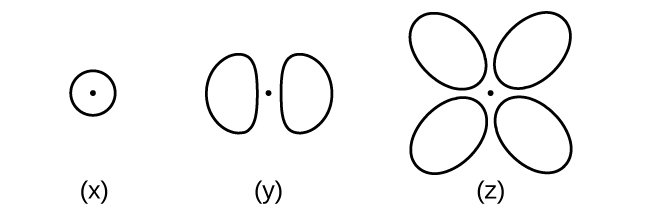(a) What is the maximum number of electrons contained in an orbital of type (x)? Of type (y)? Of type (z)?

(b) How many orbitals of type (x) are found in a shell with n = 2? How many of type (y)? How many of type (z)?

(c) Write a set of quantum numbers for an electron in an orbital of type (x) in a shell with n = 4. Of an orbital of type (y) in a shell with n = 2. Of an orbital of type (z) in a shell with n = 3.

(d) What is the smallest possible n value for an orbital of type (x)? Of type (y)? Of type (z)?

(e) What are the possible l and ml values for an orbital of type (x)? Of type (y)? Of type (z)?

12. State the Heisenberg uncertainty principle. Describe briefly what the principle implies.
13. How many electrons could be held in the second shell of an atom if the spin quantum number ms could have three values instead of just two? (Hint: Consider the Pauli exclusion principle.)
14. Which of the following equations describe particle-like behavior? Which describe wavelike behavior? Do any involve both types of behavior? Describe the reasons for your choices.

(a)$c = \lambda\nu$

(b)$E = \frac{m\nu^2}{2}$

(c)$r = \frac{n^2a_0}{Z}$

(d)$E = h\nu$

(e)$\lambda = \frac{h}{m\nu}$

15. Write a set of quantum numbers for each of the electrons with an n of 4 in a Se atom.

## Glossary

angular momentum quantum number (l)
quantum number distinguishing the different shapes of orbitals; it is also a measure of the orbital angular momentum
atomic orbital
mathematical function that describes the behavior of an electron in an atom (also called the wavefunction), it can be used to find the probability of locating an electron in a specific region around the nucleus, as well as other dynamical variables
d orbital
region of space with high electron density that is either four lobed or contains a dumbbell and torus shape; describes orbitals with l = 2. An electron in this orbital is called a d electron
electron density
a measure of the probability of locating an electron in a particular region of space, it is equal to the squared absolute value of the wave function ψ
f orbital
multilobed region of space with high electron density, describes orbitals with l = 3. An electron in this orbital is called an f electron
Heisenberg uncertainty principle
rule stating that it is impossible to exactly determine both certain conjugate dynamical properties such as the momentum and the position of a particle at the same time. The uncertainty principle is a consequence of quantum particles exhibiting wave–particle duality
magnetic quantum number (ml)
quantum number signifying the orientation of an atomic orbital around the nucleus; orbitals having different values of ml but the same subshell value of l have the same energy (are degenerate), but this degeneracy can be removed by application of an external magnetic field
p orbital
dumbbell-shaped region of space with high electron density, describes orbitals with l = 1. An electron in this orbital is called a p electron
Pauli exclusion principle
specifies that no two electrons in an atom can have the same value for all four quantum numbers
principal quantum number (n)
quantum number specifying the shell an electron occupies in an atom
quantum mechanics
field of study that includes quantization of energy, wave-particle duality, and the Heisenberg uncertainty principle to describe matter
s orbital
spherical region of space with high electron density, describes orbitals with l = 0. An electron in this orbital is called an s electron
shell
set of orbitals with the same principal quantum number, n
spin quantum number (ms)
number specifying the electron spin direction, either$+\frac{1}{2}$ or$-\frac{1}{2}$
subshell
set of orbitals in an atom with the same values of n and l
wavefunction (ψ)
mathematical description of an atomic orbital that describes the shape of the orbital; it can be used to calculate the probability of finding the electron at any given location in the orbital, as well as dynamical variables such as the energy and the angular momentum

### Solutions

Answers to Chemistry End of Chapter Exercises

1. Both models have a central positively charged nucleus with electrons moving about the nucleus in accordance with the Coulomb electrostatic potential. The Bohr model assumes that the electrons move in circular orbits that have quantized energies, angular momentum, and radii that are specified by a single quantum number, n = 1, 2, 3, …, but this quantization is an ad hoc assumption made by Bohr to incorporate quantization into an essentially classical mechanics description of the atom. Bohr also assumed that electrons orbiting the nucleus normally do not emit or absorb electromagnetic radiation, but do so when the electron switches to a different orbit. In the quantum mechanical model, the electrons do not move in precise orbits (such orbits violate the Heisenberg uncertainty principle) and, instead, a probabilistic interpretation of the electron’s position at any given instant is used, with a mathematical function ψ called a wavefunction that can be used to determine the electron’s spatial probability distribution. These wavefunctions, or orbitals, are three-dimensional stationary waves that can be specified by three quantum numbers that arise naturally from their underlying mathematics (no ad hoc assumptions required): the principal quantum number, n (the same one used by Bohr), which specifies shells such that orbitals having the same n all have the same energy and approximately the same spatial extent; the angular momentum quantum number l, which is a measure of the orbital’s angular momentum and corresponds to the orbitals’ general shapes, as well as specifying subshells such that orbitals having the same l (and n) all have the same energy; and the orientation quantum number m, which is a measure of the z component of the angular momentum and corresponds to the orientations of the orbitals. The Bohr model gives the same expression for the energy as the quantum mechanical expression and, hence, both properly account for hydrogen’s discrete spectrum (an example of getting the right answers for the wrong reasons, something that many chemistry students can sympathize with), but gives the wrong expression for the angular momentum (Bohr orbits necessarily all have non-zero angular momentum, but some quantum orbitals [s orbitals] can have zero angular momentum).

3. n determines the general range for the value of energy and the probable distances that the electron can be from the nucleus. l determines the shape of the orbital. m1 determines the orientation of the orbitals of the same l value with respect to one another. ms determines the spin of an electron.

5. (a) 2p; (b) 4d; (c) 6s

7. (a) 3d; (b) 1s; (c) 4f

9.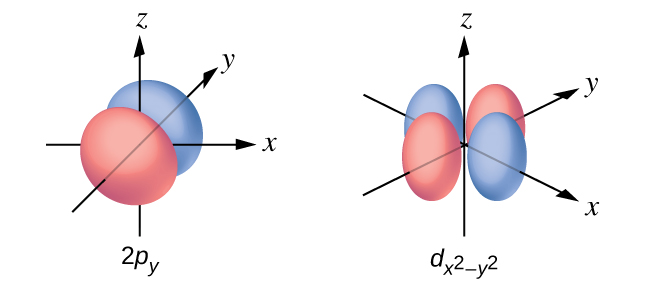11. (a) x. 2, y. 2, z. 2; (b) x. 1, y. 3, z. 0; (c) x. 4 0 0 12,12, y. 2 1 0 12,12, z. 3 2 0 12;12; (d) x. 1, y. 2, z. 3; (e) x. l = 0, ml = 0, y. l = 1, ml = –1, 0, or +1, z. l = 2, ml = –2, –1, 0, +1, +2

13. 12

15.

n l ml s
4 0 0$+\frac{1}{2}$
4 0 0$-\frac{1}{2}$
4 1 −1$+\frac{1}{2}$
4 1 0$+\frac{1}{2}$
4 1 +1$+\frac{1}{2}$
4 1 −1$-\frac{1}{2}$
Table 4.

## License6.3 Development of Quantum Theory by Rice University is licensed under a Creative Commons Attribution 4.0 International License, except where otherwise noted.